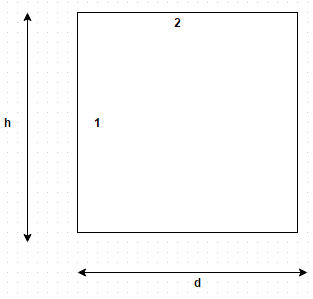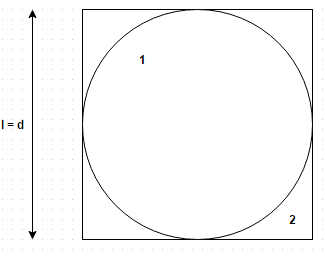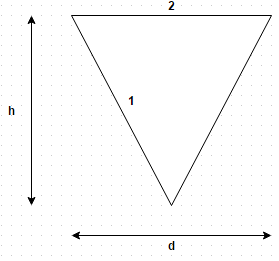Test: Shape Factor

# Test: Shape Factor

Test Description

## 11 Questions MCQ Test Heat Transfer | Test: Shape Factor

Test: Shape Factor for Chemical Engineering 2022 is part of Heat Transfer preparation. The Test: Shape Factor questions and answers have been prepared according to the Chemical Engineering exam syllabus.The Test: Shape Factor MCQs are made for Chemical Engineering 2022 Exam. Find important definitions, questions, notes, meanings, examples, exercises, MCQs and online tests for Test: Shape Factor below.
Solutions of Test: Shape Factor questions in English are available as part of our Heat Transfer for Chemical Engineering & Test: Shape Factor solutions in Hindi for Heat Transfer course. Download more important topics, notes, lectures and mock test series for Chemical Engineering Exam by signing up for free. Attempt Test: Shape Factor | 11 questions in 10 minutes | Mock test for Chemical Engineering preparation | Free important questions MCQ to study Heat Transfer for Chemical Engineering Exam | Download free PDF with solutions
 1 Crore+ students have signed up on EduRev. Have you?
Test: Shape Factor - Question 1

### Which of the followings is a wrong statement? The shape factor is equal to one

Detailed Solution for Test: Shape Factor - Question 1

For a flat or convex surface, the shape factor with respect to itself is zero. This aspect stems from the fact that for any part of flat or convex surface, one cannot see any other part of the same surface.

Test: Shape Factor - Question 2

### Establish a relation for the shape factor of cylindrical cavity with respect to itself of depth h and diameter d. The cavity is closed on its outer surface with a flat plateDetailed Solution for Test: Shape Factor - Question 2

11 + F 12 = 1 and F 21 + F 22 = 1. But F 22 = 0, so F 11 = 1 – A / A 1.

Test: Shape Factor - Question 3

### The reciprocity theorem states that Where symbols have their usual meanings

Detailed Solution for Test: Shape Factor - Question 3

It indicates the net radiation exchange can be calculated by computing one way configuration factor from either surface to the other.

Test: Shape Factor - Question 4

Two radiating surface A 1 = 6 m2 and A 2 = 4 m2 have shape factor F 12 = 0.1. Then the shape factor F 21 will be

Detailed Solution for Test: Shape Factor - Question 4

12 = A 2 F 21.

Test: Shape Factor - Question 5

What is the value of shape factor for two infinite parallel surfaces separated by a distance x?

Detailed Solution for Test: Shape Factor - Question 5

All the radiation emitted by one falls on the other so shape factor is unity.

Test: Shape Factor - Question 6

What is the shape factor of a sphere of diameter d inside a cubical box of length l = d?Detailed Solution for Test: Shape Factor - Question 6

11 + F 12 = 1 or F 12 = 1. So, F 21 = π/6.

Test: Shape Factor - Question 7

What is the shape factor of hemispherical surface closed by a plane surface of diameter d?

Detailed Solution for Test: Shape Factor - Question 7

11 + F 12 = 1 and F 21 = 1. Therefore, F 11 = 0.5.

Test: Shape Factor - Question 8

What is the shape factor of hemispherical surface closed by a plane surface of diameter d?

Detailed Solution for Test: Shape Factor - Question 8

11 + F 12 = 1 and F 21 = 1. Therefore, F 11 = 0.5.

Test: Shape Factor - Question 9

Establish a relation for shape factor for a conical cavity with respect to itself of depth h and diameter d. The cavity is closed on its outer surface with a flat plateDetailed Solution for Test: Shape Factor - Question 9

11 = 1 – A / A 1 = 1 – 2 sin α = 1 – d/ (4 h 2 + d 2½.

Test: Shape Factor - Question 10

What is the shape factor for a hemispherical bowl with respect to itself of diameter d? The cavity is closed on its outer surface with a flat plate

Detailed Solution for Test: Shape Factor - Question 10

11 = 1 – A / A = 0.5.

Test: Shape Factor - Question 11

Consider a system of concentric spheres of radius r 1 and r 2 (r 2 is greater than r 1). If r 1 = 5 cm, determine the radius r 2 if it is desired to have the value of shape factor F 21 equal to 0.6

Detailed Solution for Test: Shape Factor - Question 11

From reciprocity theorem, A 12 = A 2 F 21.

## Heat Transfer

58 videos|70 docs|85 tests
 Use Code STAYHOME200 and get INR 200 additional OFF Use Coupon Code
Information about Test: Shape Factor Page
In this test you can find the Exam questions for Test: Shape Factor solved & explained in the simplest way possible. Besides giving Questions and answers for Test: Shape Factor, EduRev gives you an ample number of Online tests for practice

## Heat Transfer

58 videos|70 docs|85 tests